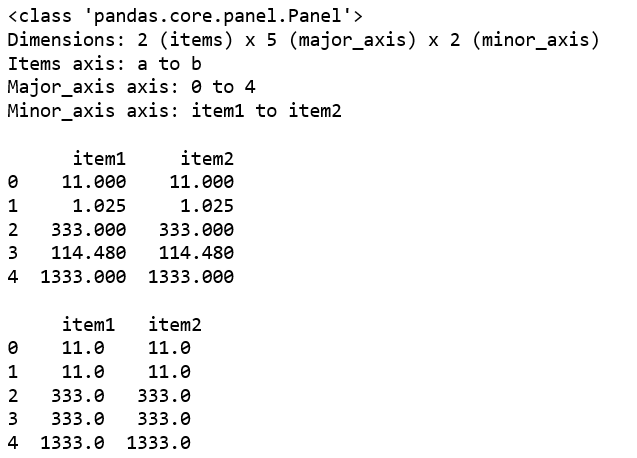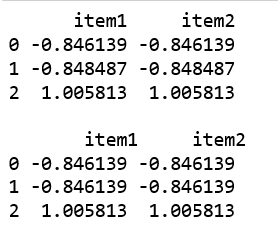# Python | Pandas Panel.cummax()

In Pandas, Panel is a very important container for three-dimensional data. The names for the 3 axes are intended to give some semantic meaning to describing operations involving panel data and, in particular, econometric analysis of panel data.

`Panel.cummax() ` function is used to returns a DataFrame or Series of the same size containing the cumulative maximum.

Syntax: Panel.cummax(axis=None, skipna=True, *args, **kwargs)

Parameters:
axis : The index or the name of the axis. 0 is equivalent to None or ‘index’.
skipna : Exclude NA/null values. If an entire row/column is NA, the result will be NA.

Returns: Cummax of DataFrame or Panel

Code #1:

 `# importing pandas module  ` `import` `pandas as pd  ` `import` `numpy as np ` ` `  `df1 ``=` `pd.DataFrame({``'a'``: [``'Geeks'``, ``'For'``, ``'geeks'``, ``'for'``, ``'real'``],  ` `                    ``'b'``: [``11``, ``1.025``, ``333``, ``114.48``, ``1333``]}) ` `                     `  `data ``=` `{``'item1'``:df1, ``'item2'``:df1} ` ` `  `# creating Panel  ` `panel ``=` `pd.Panel.from_dict(data, orient ``=``'minor'``) ` ` `  `print``(panel, ``"\n"``) ` `print``(panel[``'b'``]) ` ` `  `print``(``"\n"``, panel[``'b'``].cummax(axis ``=` `0``)) `

Output:Code #2:

 `# importing pandas module  ` `import` `pandas as pd  ` `import` `numpy as np ` ` `  `df1 ``=` `pd.DataFrame({``'a'``: [``'Geeks'``, ``'For'``, ``'geeks'``],  ` `                    ``'b'``: np.random.randn(``3``)}) ` `                     `  `data ``=` `{``'item1'``:df1, ``'item2'``:df1} ` ` `  `# creating Panel  ` `panel ``=` `pd.Panel.from_dict(data, orient ``=``'minor'``) ` `print``(panel, ``"\n"``) ` `print``(panel[``'b'``]) ` ` `  ` `  `df2 ``=` `pd.DataFrame({``'b'``: [``11``, ``12``, ``13``]}) ` `print``(``"\n"``, panel[``'b'``].cummax(axis ``=` `0``)) `

Output:My Personal Notes arrow_drop_upCheck out this Author's contributed articles.

If you like GeeksforGeeks and would like to contribute, you can also write an article using contribute.geeksforgeeks.org or mail your article to contribute@geeksforgeeks.org. See your article appearing on the GeeksforGeeks main page and help other Geeks.

Please Improve this article if you find anything incorrect by clicking on the "Improve Article" button below.

Article Tags :

Be the First to upvote.

Please write to us at contribute@geeksforgeeks.org to report any issue with the above content.